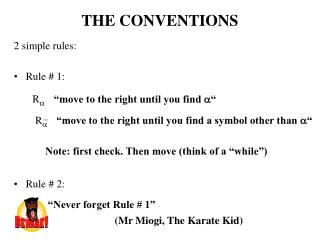DownloadDownload PresentationTHE CONVENTIONS

# THE CONVENTIONS

Télécharger la présentation## THE CONVENTIONS

- - - - - - - - - - - - - - - - - - - - - - - - - - - E N D - - - - - - - - - - - - - - - - - - - - - - - - - - -
##### Presentation Transcript

1. THE CONVENTIONS R R “move to the right until you find “ “move to the right until you find a symbol other than “ 2 simple rules: • Rule # 1: • Rule # 2: Note: first check. Then move (think of a “while”) “Never forget Rule # 1” (Mr Miogi, The Karate Kid)

2. Input and Output of Turing Machines When constructing Turing machines we have to establish conventions: For example, the Turing machine ( = { , a, b, , }): … Does the following: when starting with a configuration of the form (s, w), where w  ( - {, , })* then the final configuration is (h, ww).

3. Acceptance in Previous Machines Machine Configuration Acceptance if q: final state, w = e (q,w) Finite automata q: final state, w =e  = e Pushdown automata (q,w,) Pushdown automata accepting by empty stack w =e,  = e (q,w,) These machines are used to compute if a word belongs to a language

4. Input and Output of Turing Machines (2) … a a   b a state: s ( - {, })* … b a   a b state: h ( - {, })* 0 Idea: Input: Output:

5. Functions (Again) This definition says that 2 things should happen: Second, that each element in A has a unique representative in B First, that “no one (in A) is left behind” B A B A a a d d Definition. A function, f, from A to B is a relation such that for every a  A there is one and only one b  A with f(a) = b Example: transitions in Turing machines

6. Functions (Again – Part 2) From the two restrictions, the first can be easily overcome: A Dom(f) = {a  A : there is a b  B with f(a) = b} One can restrict f to be from Dom(f) to B. In this situation one speaks of a partial function Example: f from Real to Real defined as f(x) = 1/x. Dom(f) = Real - {0} (Thus f is a partial function)

7. Input and Output of Turing Machines (3) Definition. Let: • M = (S, , , s, H) be a Turing machine • 0 =  - {, } • Suppose that M starts with (s,w) with w  0* If the machine ends in a configuration (h,u) with u  0* and h  H, then: • u is referred to as the output of M and write M(w) = u • We can view M as a function f (from 0* into 0*), with f(w) = u. We say that M computes f. • If M does not end for a w’  0*, then we write M(w’)

8. Turing-Computable Functions Definition. A function f: 0*  0* is said to be Turing computable if there exists a Turing machine M that computes it (i.e., for each w  dom(f), f(w)=M(w)) and dom(f) = 0*. If dom(f)is an strict subset of 0* (i.e., dom(f)  0* and dom(f)  0*), we say that f is partial Turing computable

9. Example of a Turing-Computable Function f(w) = wa where a is a fixed character in 0 and w  0*

10. Example of a Turing-Computable Function (2) Let M be the machine that terminates in the configuration (h, ww) when starting with (s, w) (see Slide # 2) M f(w) = ww where and w  0* …

11. Computing (Deciding) Languages Definition. Let L be a language in 0* we define the characteristic function, L, as follows: • L(w) = 1, if w  L • L(w) = 0, otherwise Definition. A language L is Turing-computable or decidable if its characteristic function, L, is Turing-computable

12. Regular Languages are Turing-Computable (1) Steps to obtain a Turing machine accepting a regular language: • Let L be a regular language on alphabet {a,b} • Let A be a deterministic automata accepting L • It is easy to simulate A with a Turing machine ML: • ((p,a),q) ((p,a),(q,)) For every favorable state f in A, add the transition: ((f,),(h,1))

13. Regular Languages are Turing-Computable (2) 1 M1 1 M0 Use the machine ML as follows: ML This Turing machine recognizes the language L Every regular language is Turing-computable (decidable)

14. Homework • 4.9, 4.10, See slide # 10